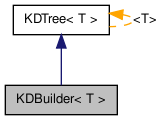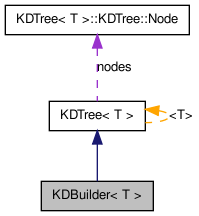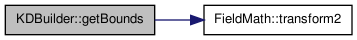KDBuilder< T > Class Template Reference

Derived type used to construct KDTree instances (makeTree static method). More...

#include <kdTree.h>

Inheritance diagram for KDBuilder< T >:[legend]
Collaboration diagram for KDBuilder< T >:[legend]

List of all members.

Classes

struct  BoundsExpander
Expands a valid bounding box to contain a point (one coordinate at once). More...
struct  NewBounds
Creates bounds containing one value. More...

Public Types

typedef int(KDBuilder::* CoordChooser )(int nodeIndex, int *beginIDs, int *endIDs, int depthLeft) const
Type for a method that chooses which coordinate to split.

Public Member Functions

int choosePrecise (int nodeIndex, int *beginIDs, int *endIDs, int) const
CoordChooser choosing the longest coordinate of the bounding box of the current interval.
int chooseFast (int, int *, int *, int depthLeft) const
CoordChooser choosing the coordinate only according to the depth.
int chooseRand (int, int *, int *, int) const
CoordChooser choosing a random coordinate.
int chooseApprox (int nodeIndex, int *, int *, int depthLeft) const
CoordChooser - like choosePrecise, but doesn't compute the real bounding box, only approximates it by splitting the parent's box (a little less accurate, but much faster).

Static Public Member Functions

static TreemakeTree (const T *data, int length, int count, CoordChooser chooser)
Builds a KDTree from count vectors of length length stored in data, splitting the nodes by chooser CoordChooser.

Protected Member Functions

void getBounds (Bounds boundsRes) const
Computes the bounding box of count vectors with length length stored in data.
void getBounds (const int *beginIDs, const int *endIDs, Bounds boundsRes) const
Like getBounds, but it only works on vectors with indices from [beginIDs;endIDs) instead of [0,count), boundsRes should be preallocated to store the result.
void buildNode (int nodeIndex, int *beginIDs, int *endIDs, int depthLeft)
Recursively builds node nodeIndex and its subtree of depth depthLeft (including leaves), operates on data data in the range [beginIDs,endIDs).

Detailed Description

template<class T> class KDBuilder< T >

Derived type used to construct KDTree instances (makeTree static method).

Definition at line 277 of file kdTree.h.

Member Function Documentation

template<class T >
 void KDBuilder< T >::getBounds ( Bounds boundsRes ) const [inline, protected]

Computes the bounding box of count vectors with length length stored in data.

The vectors in data are stored linearly, boundsRes should be preallocated

Definition at line 322 of file kdTree.h.

References FieldMath::transform2().

Here is the call graph for this function:The documentation for this class was generated from the following file:

Generated on Thu Aug 6 22:33:14 2009 for Fractal Image Compressor by1.5.8